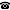# 2019 seminar talk: Forcing against bounded arithmetic

Talk held by Moritz Müller (Universitat Politècnica de Catalunya, Barcelona, Spain) at the KGRC seminar on 2019-01-17.

### Abstract

We study the following problem. Given a nonstandard model of arithmetic we want to expand it by a binary relation that does something prohibitive, e.g. violates the pigeonhole principle in the sense that it is the graph of a bijection from $n+1$ onto $n$ for some (nonstandard) $n$ in the model. The goal is to do so while preserving as much as possible of true arithmetic. More precisely, we want the expansion to model the least number principle for a class of formulas as large as possible. The problem is of central importance in bounded arithmetic and propositional proof complexity. It is not well understood. The talk describes a general method of forcing to produce such expansions.

Slides for this talk are available.

Kurt Gödel Research Center for Mathematical Logic. Währinger Straße 25, 1090 Wien, Austria.+43-1-4277-50501. Last updated: 2010-12-16, 04:37.